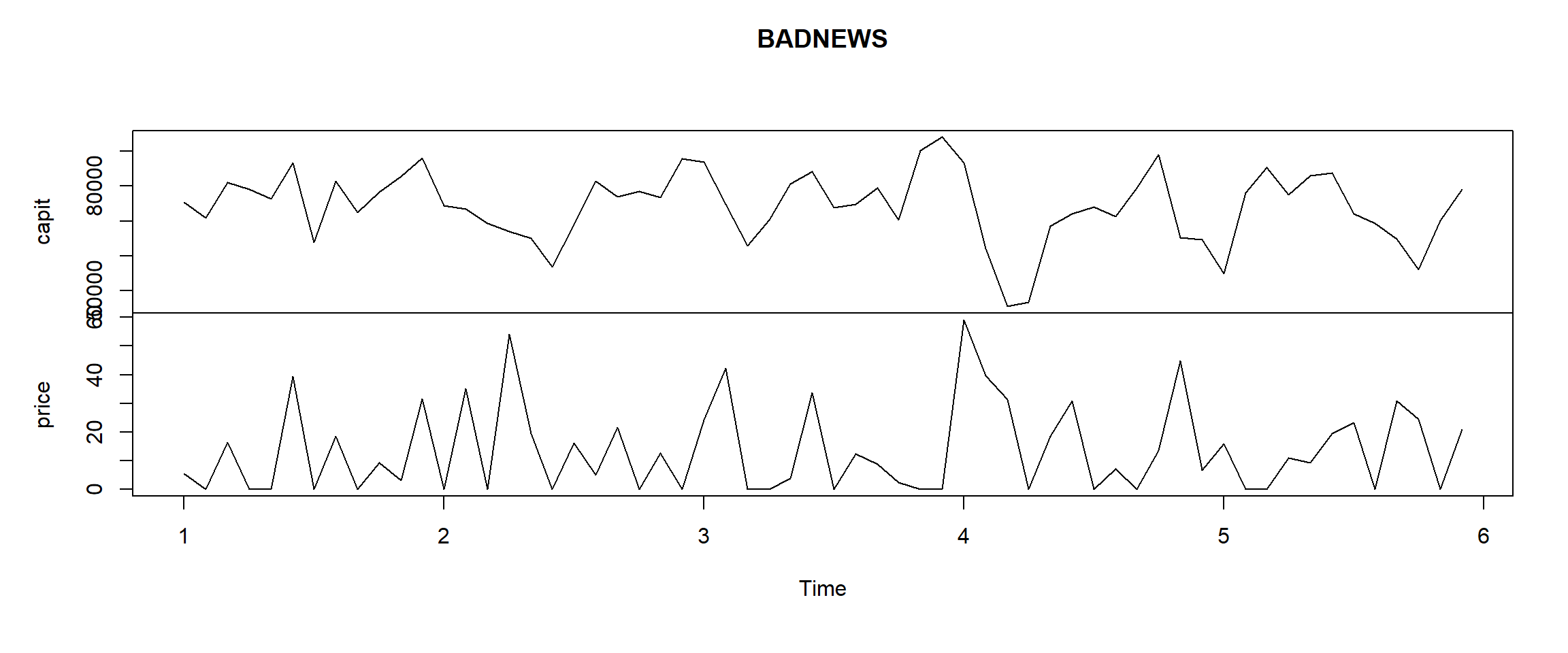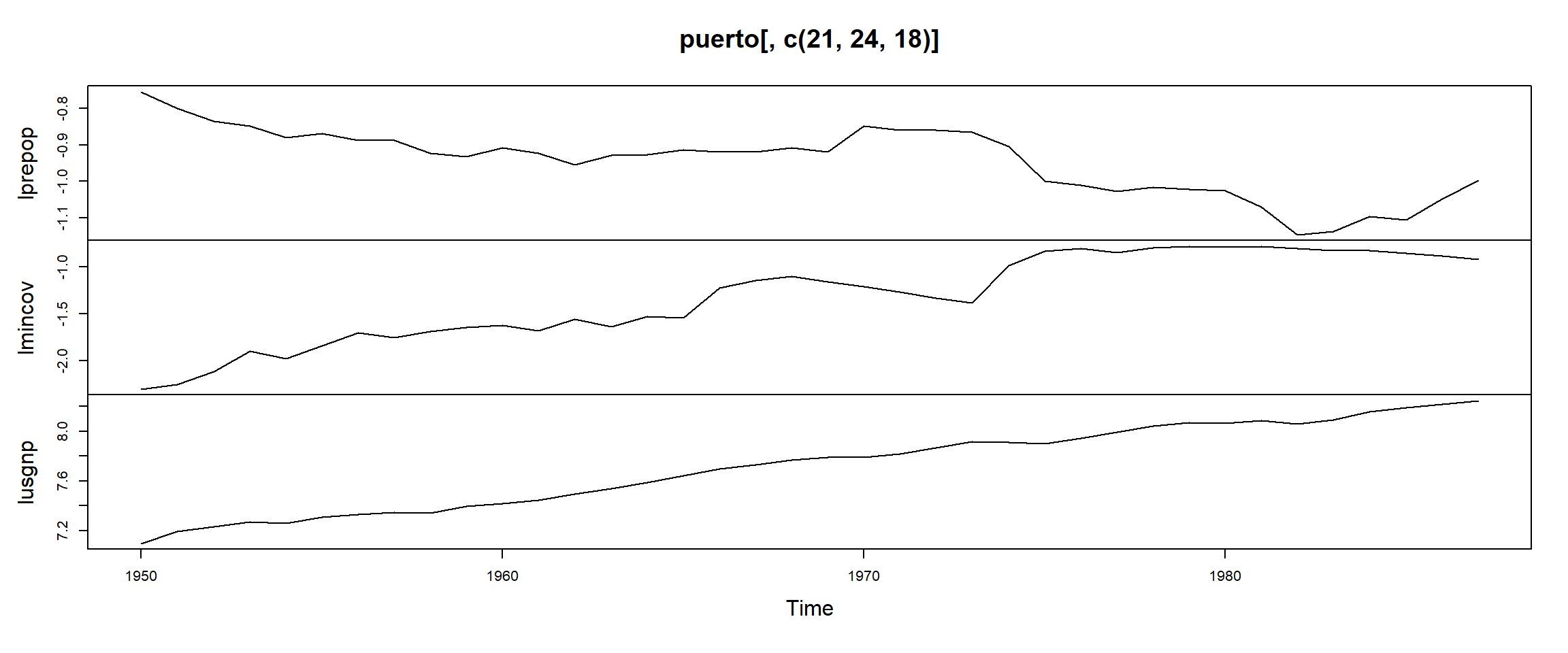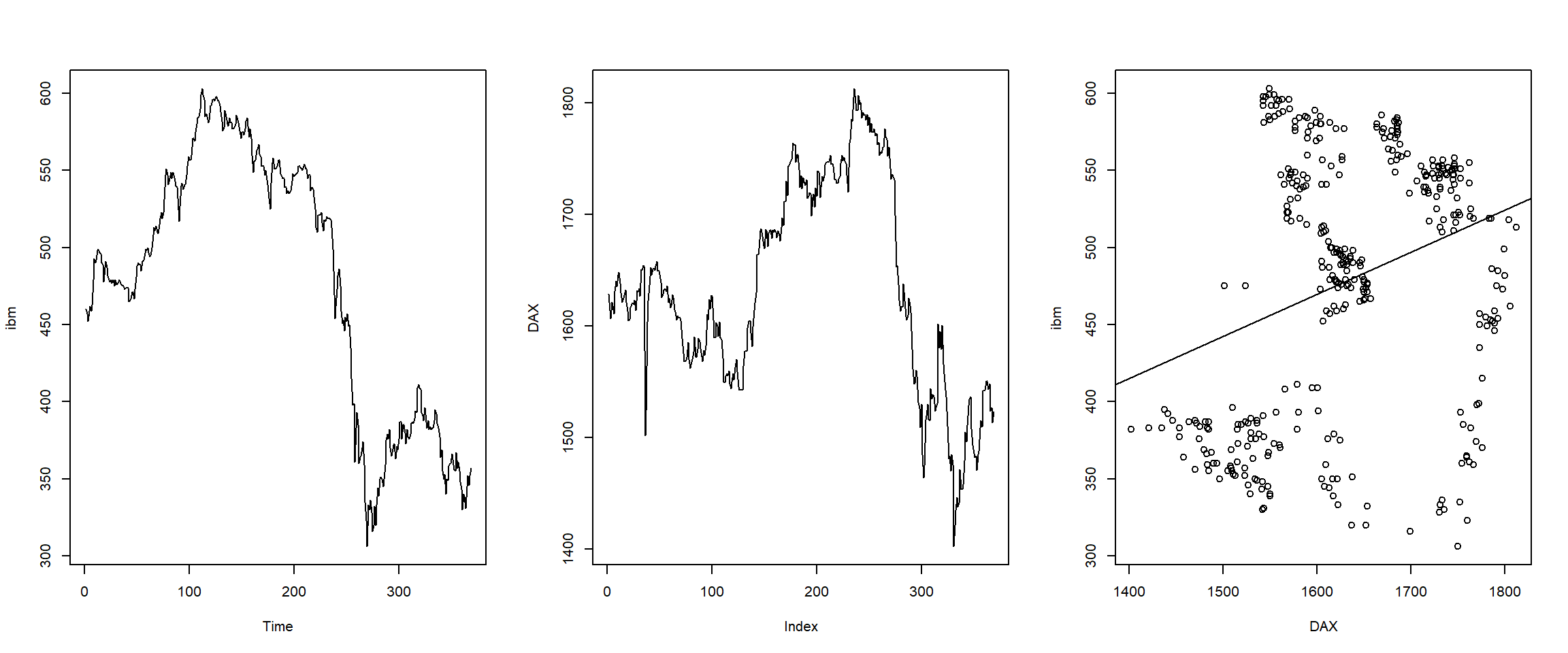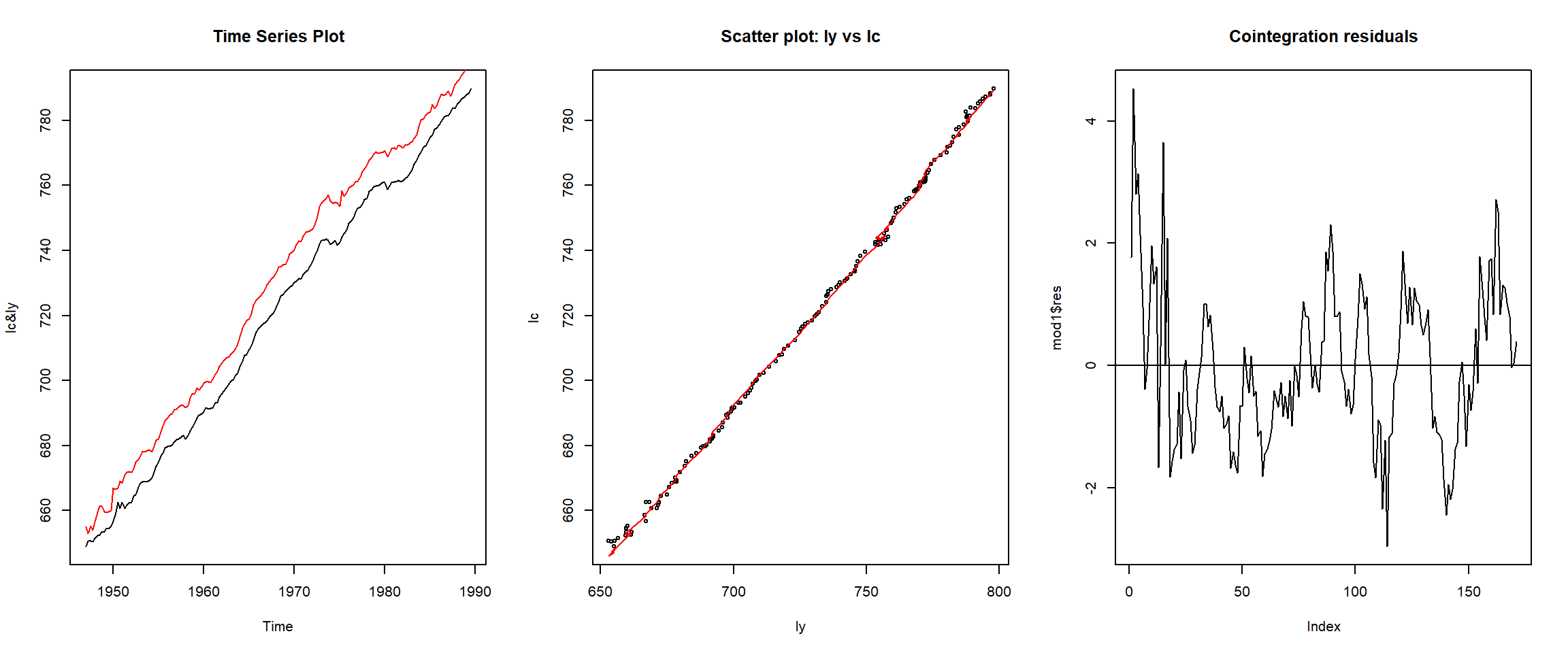# General examples

## Regression with Time Lags: Autoregressive Distributed Lag Models

We shall re-do the example from the lecture slides.

Say we have data collected on a monthly basis over five years (i.e., 60 months) on the following variables:

• Y market capitalization of Company B (in $$\$$ ’000)
• X the price of oil (dollars per barrel) above the benchmark price
suppressPackageStartupMessages({
suppressWarnings({
library(gdata)
})
})
txt1 <- "http://uosis.mif.vu.lt/~rlapinskas/(data%20R&GRETL/"
tmp = tempfile(fileext = ".xls")
destfile = tmp, mode = "wb")
#Read it as an excel file
from = c("Y", "X"),
to = c("capit", "price"), info = FALSE)
BADNEWS <- ts(BADNEWS, freq = 12)
plot.ts(BADNEWS)Since this is time series data and it is likely that previous months news about the oil price will affect current market capitalization, it is necessary to include lags of X in the regression. Below are present OLS estimates of the coefficients in a distributed lag model in which market capitalization is allowed to depend on present news about the oil price and news up to $$q_{max} = 4$$ months ago. That is: $capit_t = \alpha + \beta_0 price_t + \beta_1 price_{t-1} + ... + \beta_4 price_{t-4} + \epsilon_t$

suppressPackageStartupMessages({library(dynlm)})
mod.L4 <- dynlm(capit ~ L(price, 0:4), data = BADNEWS)
round(summary(mod.L4)$coef, 4) ## Estimate Std. Error t value Pr(>|t|) ## (Intercept) 91173.3150 1949.8502 46.7591 0.0000 ## L(price, 0:4)0 -131.9943 47.4361 -2.7826 0.0076 ## L(price, 0:4)1 -449.8597 47.5566 -9.4595 0.0000 ## L(price, 0:4)2 -422.5183 46.7778 -9.0324 0.0000 ## L(price, 0:4)3 -187.1041 47.6409 -3.9274 0.0003 ## L(price, 0:4)4 -27.7710 47.6619 -0.5827 0.5627 Just looking at the coefficient values, what can we conclude about the effect of news about the oil price on Company B’s market capitalization? Increasing the oil price by one dollar per barrel in a given month is associated with: 1. An immediate reduction in market capitalization of$ 131’994, ceteris paribus.
2. A reduction in market capitalization of $449’860 on month later, ceteris paribus. and so on. To provide some intuition about what the ceteris paribus condition implies in this context, note that, for example, we can also express the second statement as: ‘Increasing the oil price by one dollar in a given month will tend to reduce the market capitalization in the following month by$ 449’860, assuming that no other change in the oil price occurs’.

Since the p-value corresponding to the explanatory variable $$price_{t-4}$$ is greater than 0.05, we cannot reject the null hypothesis that $$\beta_4 = 0$$ at the 5% level of significance. Accordingly we drop this variable from the model and re-estimate the lag length equal to 3, yielding the following results:

mod.L3 <- dynlm(capit ~ L(price, 0:3), data = BADNEWS)
round(summary(mod.L3)coef, 4) ## Estimate Std. Error t value Pr(>|t|) ## (Intercept) 90402.2210 1643.1828 55.0165 0.0000 ## L(price, 0:3)0 -125.9000 46.2405 -2.7227 0.0088 ## L(price, 0:3)1 -443.4918 45.8816 -9.6660 0.0000 ## L(price, 0:3)2 -417.6089 45.7332 -9.1314 0.0000 ## L(price, 0:3)3 -179.9043 46.2520 -3.8896 0.0003 The p-value for testing $$\beta_3 = 0$$ is 0.0003, which is much less than 0.05. We therefore conclude that the variable $$price_{t-3}$$ does indeed belong in the distributed lag model. Hence $$q = 3$$ is the lag length we select for this model. In a formal report, we would present this table of results or the equation: $capit_t = 90402.22 -125.9 price_t -443.49 price_{t-1} -417.61 price_{t-2} -179.90 price_{t-3}$ Since these results are similar to those discussed above, we will not repeat their interpretation. ## Short-run and Long run multipliers In general, considering the following model: $Y_t = \alpha + \phi Y_{t-1} + \beta_0 X_t + \beta_1 X_{t-1} + \epsilon_t, \quad 0 < \phi < 1$ • The short-run multiplier can be calculated by taking the partial derivatives: $\dfrac{\partial Y_t}{\partial X_t} = \beta_0$ Which shows that an increase in $$X$$ with one unit has an immediate impact on $$Y$$ of $$\beta_0$$ units. • The long-run multiplier (or equilibrium multiplier): In order to calculate the long-run multiplier, we need to see how a one-unit increase in $$X$$ at time $$t$$ affects $$Y$$ as $$t$$ increases. The effect after one period is: $\dfrac{\partial Y_{t+1}}{\partial X_t} = \phi \dfrac{\partial Y_t}{\partial X_t} + \beta_1 = \phi \beta_0 + \beta_1$ Similarly, after two periods: $\dfrac{\partial Y_{t+2}}{\partial X_t} = \phi \dfrac{\partial Y_{t+1}}{\partial X_t} = \phi (\phi \beta_0 + \beta_1)$ and so on. This shows that after the first period, the effect is decreasing if $$|\phi| < 1$$. Imposing this so-called stability condition (i.e. $$|\phi| < 1$$) allows us to determine the long-run effect of a permanent unit change in $$X_t$$. $\beta_0 + (\phi \beta_0 + \beta_1) + \phi (\phi \beta_0 + \beta_1) + ... =\beta_0 + (1 + \phi + \phi^2 +...)(\phi\beta_0 + \beta_1) = \dfrac{\beta_0 + \beta_1}{1 - \phi}$ This says that if the unit increase in $$X_t$$ is permanent, the expected long-run permanent cumulative change in $$Y$$ is given by $$\dfrac{\beta_0 + \beta_1}{1 - \phi}$$. Considerations Note that we can calculate the symbolic expressions of the partial derivatives in R! Unfortunately, we cannot combine the expressions in an intuitive way (as we can in Python). We begin by specifying the equations: expr_t0 = quote(phi*Y.lag.1 + b0*X + b1*X.lag.1) expr_t1 = substitute(phi * expr_t0 + beta_0 * X_t_plus_1 + beta_1 * X, list(expr_t0 = expr_t0)) expr_t2 = substitute(phi * expr_t1 + beta_0 * X_t_plus_2 + beta_1 * X_t_plus_1, list(expr_t1 = expr_t1)) print("Y_{t}:") print(expr_t0) print(paste0(rep("-", 100), collapse= "")) print("Y_{t+1}:") print(expr_t1) print(paste0(rep("-", 100), collapse= "")) print("Y_{t+2}:") print(expr_t2) print(paste0(rep("-", 100), collapse= "")) #print(paste0("Incorrect expression example: ", expression(phi*expr_t0 + b0*X.1 + b1*X))) ##  "Y_{t}:" ## phi * Y.lag.1 + b0 * X + b1 * X.lag.1 ##  "----------------------------------------------------------------------------------------------------" ##  "Y_{t+1}:" ## phi * (phi * Y.lag.1 + b0 * X + b1 * X.lag.1) + beta_0 * X_t_plus_1 + ## beta_1 * X ##  "----------------------------------------------------------------------------------------------------" ##  "Y_{t+2}:" ## phi * (phi * (phi * Y.lag.1 + b0 * X + b1 * X.lag.1) + beta_0 * ## X_t_plus_1 + beta_1 * X) + beta_0 * X_t_plus_2 + beta_1 * ## X_t_plus_1 ##  "----------------------------------------------------------------------------------------------------" Then we can calculate the partial derivative expressions: #Partial derivative of Y_t with respect to X_t D(expr_t0, "X") #Partial derivative of Y_{t+1} with respect to X_t D(expr_t1, "X") #Partial derivative of Y_{t+2} with respect to X_t D(expr_t2, "X") ## b0 ## phi * b0 + beta_1 ## phi * (phi * b0 + beta_1) We can even evaluate the expression for specific values: val <- D(expression(phi*Y.lag.1 + b0*X + b1*X.lag.1), "X") eval(val, envir = list(b0 = 1)) val2 <- D(expression(phi*(phi*Y.lag.1 + b0*X + b1*X.lag.1) + b0*X.1 + b1*X), "X") eval(val2, envir = list(b0 = 1, b1 = 0.5, phi = -0.1)) ##  1 ##  0.4 ## Time Series Regression when Both $$Y$$ and $$X$$ are Trend Stationary: Spurious regression Data contains the yearly data on Puerto Rican employment rate, minimum wage and other variables. suppressPackageStartupMessages({ suppressWarnings({ library(readxl) library(gdata) }) }) txt1 <- "http://uosis.mif.vu.lt/~rlapinskas/(data%20R&GRETL/" txt2 <- "prmin.data.xls" tmp = tempfile(fileext = ".xls") #Download the file download.file(url = paste0(txt1, txt2), destfile = tmp, mode = "wb") #Read it as an excel file puerto <- ts(read_excel(path = tmp, col_names = FALSE), start=1950, freq = 1) ## New names: ## *  -> ...1 ## *  -> ...2 ## *  -> ...3 ## *  -> ...4 ## *  -> ...5 ## * … and 20 more problems ## Warning in data.matrix(data): NAs introduced by coercion ## Warning in data.matrix(data): NAs introduced by coercion colnames(puerto) <- c("year","avgmin","avgwage","kaitz","avgcov","covt","mfgwage", "prdef","prepop","prepopf","prgnp","prunemp","usgnp","tt", "post74", "lprunemp","lprgnp","lusgnp","lkaitz","lprun_1", "lprepop","lprep_1", "mincov","lmincov","lavgmin") We will estimate the following model: \begin{aligned} lprepop_t &= \beta_0 + \beta_1 lmincov_t + \beta_2 lusgnp_t + \epsilon_t \end{aligned} where • $$lprepop = log(PR employ/popul ratio)$$ • $$lmincov = log((avgmin/avgwage)*avgcov)$$ • $$lusgnp = log(US GNP)$$ avgmin is the average minimum wage, avgwage is the average overall wage, and avgcov is the average coverage rate (the proportion of workers actually covered by the minimum wage law). plot(puerto[,c(21,24,18)])All the variables have a trend close to linear (accurate analysis would require to test for unit root first!). round(summary(lm(lprepop ~ lmincov + lusgnp, data = puerto))coefficients, 4)
##             Estimate Std. Error t value Pr(>|t|)
## (Intercept)  -1.0544     0.7654 -1.3776   0.1771
## lmincov      -0.1544     0.0649 -2.3797   0.0229
## lusgnp       -0.0122     0.0885 -0.1377   0.8913

Thus, if the minimum wage increases then employment declines which matches classical economics. On the other hand, the GNP seems to be not significant but we shall test the claim right now:

round(summary(lm(lprepop~lmincov+lusgnp+tt,data=puerto))$coefficients, 4) ## Estimate Std. Error t value Pr(>|t|) ## (Intercept) -8.6963 1.2958 -6.7113 0e+00 ## lmincov -0.1687 0.0442 -3.8126 6e-04 ## lusgnp 1.0573 0.1766 5.9860 0e+00 ## tt -0.0324 0.0050 -6.4415 0e+00 Here, we interpret the coefficient of lusgnp as follows: if usgnp raises 1% more then, it should according to its long run trend, prepop will raise extra 1.057%. The above regression is equivalent to this (rewritten initial equation in the form of deviations from the trend): round( summary(lm(lm(lprepop~tt)$res~lm(lmincov~tt)$res+lm(lusgnp~tt)$res,data=puerto))$coefficients, 4) ## Estimate Std. Error t value Pr(>|t|) ## (Intercept) 0.0000 0.0061 0.0000 1e+00 ## lm(lmincov ~ tt)$res  -0.1687     0.0436 -3.8683    5e-04
## lm(lusgnp ~ tt)$res 1.0573 0.1741 6.0734 0e+00 # alternatively in a more readable format: tilde_lprepop <- lm(lprepop~tt, data=puerto)$res
tilde_lmincov <- lm(lmincov~tt, data=puerto)$res tilde_lusgnp <- lm(lusgnp~tt, data=puerto)$res
round(
summary(lm(tilde_lprepop ~ tilde_lmincov + tilde_lusgnp))$coefficients, 4) ## Estimate Std. Error t value Pr(>|t|) ## (Intercept) 0.0000 0.0061 0.0000 1e+00 ## tilde_lmincov -0.1687 0.0436 -3.8683 5e-04 ## tilde_lusgnp 1.0573 0.1741 6.0734 0e+00 We can see that accounting for a time effect determines the significance of the exogenous variables in our model. ## Time Series Regression when Y and X Have Unit Roots: Spurious Regression Let us analyze daily IBM stock prices spanning May 17, 1961 to November 2, 1962 (369 days in all) and daily closing prices of German DAX index starting at the 130th day of 1991. suppressPackageStartupMessages({ suppressWarnings({ library(dynlm) library(waveslim) library(datasets) }) }) #?ibm data(ibm) #?EuStockMarkets data(EuStockMarkets) DAX=EuStockMarkets[1:369, 1] par(mfrow = c(1,3)) plot(ibm); plot(DAX, type = "l") iD = lm(ibm ~ DAX) plot(DAX, ibm); abline(iD)round(summary(iD)$coefficients, 4)
##             Estimate Std. Error t value Pr(>|t|)
## (Intercept)  31.9930    74.0349  0.4321   0.6659
## DAX           0.2735     0.0453  6.0403   0.0000

Though, because of their nature, ibm and DAX should not have any relationship, the coefficient of regression is very significant. This is an example of spurious regression which can be explained through the fact that the errors of the model have a unit root.

The following should be carried out:

1. Establish that ibm has a unit root
2. Establish that DAX has a unit root
3. Verify that the errors of the ID model have a unit root

The (1) and (2) can be carried out in the same manner as in the previous lectures. Regarding (3):

iD.res=ts(iD$res) round(summary(dynlm(d(iD.res)~L(iD.res)+time(iD.res)))$coefficients, 4)
##              Estimate Std. Error t value Pr(>|t|)
## (Intercept)    1.8987     1.0885  1.7443   0.0820
## L(iD.res)     -0.0134     0.0071 -1.8838   0.0604
## time(iD.res)  -0.0113     0.0054 -2.1066   0.0358

Recall that the unit root hypothesis $$H_0$$ claims that the coefficient at L(iD.res) equals zero. In this case of testing for a unit root in errors, we have a further complication: here the t-statistics has not the Dickey-Fuller, but the Engle-Granger distribution whose critical values are given in this table:

No. of variables
(included Y)

1%
Significance level
5%

10%
2 -3.90 -3.34 -3.04
3 -4.29 -3.74 -3.45
4 -4.64 -4.10 -3.81
5 -4.96 -4.42 -4.13

In order to test the cointegration of these two variables note that the t-statistics equals -1.884 which is closer to 0 than -3.34, therefore there is no ground to reject $$H_0$$. Thus the errors have a unit root or, in other words, ibm and DAX are not cointegrated and the strong regression bonds are only spurious.

## Time Series Regression when $$Y$$ and $$X$$ Have Unit Roots: Cointegration

It is well known that many economic time series are DS and therefore the regression for levels is often misleading (the standard Student or Wald tests provide wrong p-values). On the other hand, if these DS series are cointegrated, the OLS method is OK.

Recall that if Y and X have unit roots (i.e., are nonstationary), but some linear combination $$Y_t - \alpha - \beta X_t$$ is (trend) stationary, then we say that Y and X are cointegrated.

In order to establish cointegration, we estimate unknown coefficients $$\alpha$$ and $$\beta$$ by means of OLS and then test whether the errors of the model $$Y_t = \alpha+ \beta X_t (+ \gamma t) + \epsilon_t$$ have a unit root (as always, respective test is applied to the residuals $$e_t = \hat{\epsilon}_t = Y_t - \hat{\alpha} - \hat{\beta} X_t (-\hat{\gamma}t)$$).

The dataset contains quarterly data, 1947:01 - 1989:03, where

• lc - logarithms of the real quarterly personal expenditure (consumption)
• ly - logarithms of the real quarterly aggregated disposable income
txt1 <- "http://uosis.mif.vu.lt/~rlapinskas/(data%20R&GRETL/"
txt2 <- "hamilton.txt"
#Read it as an excel file
ham <- read.csv(paste0(txt1, txt2), header = TRUE, sep = " ")[, -1]
lc=ts(ham[,1],start=1947,freq=4)
ly=ts(ham[,2],start=1947,freq=4)

The cointegration equation:

mod1 = lm(lc ~ ly + time(ly)) # cointegration equation (time included)
round(summary(mod1)$coefficients, 4) ## Estimate Std. Error t value Pr(>|t|) ## (Intercept) -2003.8047 152.3933 -13.1489 0 ## ly 0.6557 0.0253 25.9632 0 ## time(ly) 1.1410 0.0867 13.1541 0 par(mfrow=c(1,3)) plot(lc, ylab = "lc&ly", main = "Time Series Plot") lines(ly, col=2) plot(ly, lc, main = "Scatter plot: ly vs lc") lines(as.numeric(ly),mod1$fit,col = 2)
plot(mod1$res, type = "l", main = "Cointegration residuals");abline(0,0)It is easy to verify that both series have unit roots and their final models are: suppressPackageStartupMessages({ suppressWarnings({ library(dynlm) library(urca) }) }) #Test for unit root in lc mod2c = dynlm(d(lc) ~ L(lc) + L(d(lc),1:5) + time(lc)) #Continue the procedure until only the significant variables remain... mod2c = dynlm(d(lc) ~ L(lc) + L(d(lc), 1:2) + time(lc)) #Do the same for ly mod4y = dynlm(d(ly) ~ L(ly) + L(d(ly), 1:4)) #Model output round(summary(mod2c)$coefficients, 4)
##                 Estimate Std. Error t value Pr(>|t|)
## (Intercept)    -307.4920   129.8662 -2.3678   0.0191
## L(lc)            -0.0520     0.0218 -2.3918   0.0179
## L(d(lc), 1:2)1    0.0655     0.0759  0.8628   0.3895
## L(d(lc), 1:2)2    0.2395     0.0759  3.1571   0.0019
## time(lc)          0.1755     0.0739  2.3752   0.0187
round(summary(mod4y)$coefficients, 4) ## Estimate Std. Error t value Pr(>|t|) ## (Intercept) 2.8023 1.4982 1.8705 0.0632 ## L(ly) -0.0025 0.0020 -1.2216 0.2236 ## L(d(ly), 1:4)1 0.0081 0.0764 0.1061 0.9157 ## L(d(ly), 1:4)2 -0.0615 0.0753 -0.8163 0.4155 ## L(d(ly), 1:4)3 0.0828 0.0753 1.1005 0.2728 ## L(d(ly), 1:4)4 -0.2104 0.0737 -2.8558 0.0049 The $$t$$-statistic of the coefficient of L(lc) is $$-2.392 > -3.45$$ and the $$t$$-statistic of L(ly) is $$-1.222 > -2.89$$. In both cases we do not reject the null hypothesis that a unit root is present in each of these time series. Recall that these models can also be created ´automatically´ (if using selectlags=´AIC´) with the ur.df function from the urca package: summary(ur.df(lc, type = "trend", lags = 13,selectlags = "AIC")) ## ## ############################################### ## # Augmented Dickey-Fuller Test Unit Root Test # ## ############################################### ## ## Test regression trend ## ## ## Call: ## lm(formula = z.diff ~ z.lag.1 + 1 + tt + z.diff.lag) ## ## Residuals: ## Min 1Q Median 3Q Max ## -3.0917 -0.3565 0.0356 0.4626 2.7845 ## ## Coefficients: ## Estimate Std. Error t value Pr(>|t|) ## (Intercept) 32.93396 14.24322 2.312 0.02211 * ## z.lag.1 -0.04993 0.02203 -2.266 0.02483 * ## tt 0.04185 0.01869 2.239 0.02658 * ## z.diff.lag1 0.05440 0.07822 0.695 0.48781 ## z.diff.lag2 0.25265 0.07819 3.231 0.00151 ** ## --- ## Signif. codes: 0 '***' 0.001 '**' 0.01 '*' 0.05 '.' 0.1 ' ' 1 ## ## Residual standard error: 0.7771 on 152 degrees of freedom ## Multiple R-squared: 0.08556, Adjusted R-squared: 0.06149 ## F-statistic: 3.555 on 4 and 152 DF, p-value: 0.008376 ## ## ## Value of test-statistic is: -2.2665 12.4227 2.6088 ## ## Critical values for test statistics: ## 1pct 5pct 10pct ## tau3 -3.99 -3.43 -3.13 ## phi2 6.22 4.75 4.07 ## phi3 8.43 6.49 5.47 Or even easier with the tseries package: tseries::adf.test(lc, alternative = "stationary") ## Registered S3 method overwritten by 'xts': ## method from ## as.zoo.xts zoo ## Registered S3 method overwritten by 'quantmod': ## method from ## as.zoo.data.frame zoo ## ## Augmented Dickey-Fuller Test ## ## data: lc ## Dickey-Fuller = -2.0851, Lag order = 5, p-value = 0.5408 ## alternative hypothesis: stationary tseries::adf.test(ly, alternative = "stationary") ## ## Augmented Dickey-Fuller Test ## ## data: ly ## Dickey-Fuller = -1.2893, Lag order = 5, p-value = 0.873 ## alternative hypothesis: stationary In both cases, p-value > 0.05, so we have no grounds to reject the null hypothesis $$H_0: \rho = 0$$ of a unit root. Having determined that the series is integrated, we want to test whether they are cointegrated. We want to test whether the errors from the model: $$lc_t = \alpha + \beta ly_t + \gamma t + \epsilon_t$$ make a stationary process, i.e. the errors do not have a unit root. The arguments of the function dynlm must be a time series. We will try two different models cointegration models - one with a trend and one without. We convert the residuals of the cointegrated models to time series objects: cy.res.no.trend <- ts(lm(lc ~ ly)$res, start = 1947, frequency = 4)
cy.res.trend    <- ts(lm(lc ~ ly + time(lc))\$res, start = 1947, frequency = 4)

We want to test whether $$|\phi| < 0$$ in $$cy.res_t = \phi cy.res_{t-1} + \epsilon_t$$. However, if the errors of this model do not constitute WN, the standard OLS procedure gives the erroneous estimate of $$\phi$$. One approach is to replace the AR(1) model by an AR(p) model. Another approach is to use the AR(1) model, but to take into account the fact that the errors are not a WN (this could be done by the Phillips-Ouliaris test).

### 1st method

This method is also called the Engle-Granger method, it tests the unit root in errors hypothesis. However, the OLS procedure proposes the coefficients to the cointegration equation such that the variance of the residuals is minimum, thus the residuals are ¥too stationary´. In other words, the null hypothesis for a unit root will be rejected too often.

This is why we use other critical values to test the null hypothesis $$H_0: \rho = 0$$ and $$\Delta e_t = \rho e_{t-1} + \gamma_1 \Delta e_{t-1} + ... + \gamma_{p-1} \Delta e_{t-p+1} + w_t$$.

The asymptotic 5% critical values for residual unit root tests for cointegration in $Y_t = \alpha + \beta X_t + \gamma_t + \epsilon_t$

No. of X’s in the
right-hand-side of eq.
No deterministic
terms in eq.
Only constant
in eq.
Constant and
Trend in eq.
1 -2.76 -3.37 -3.80
2 -3.27